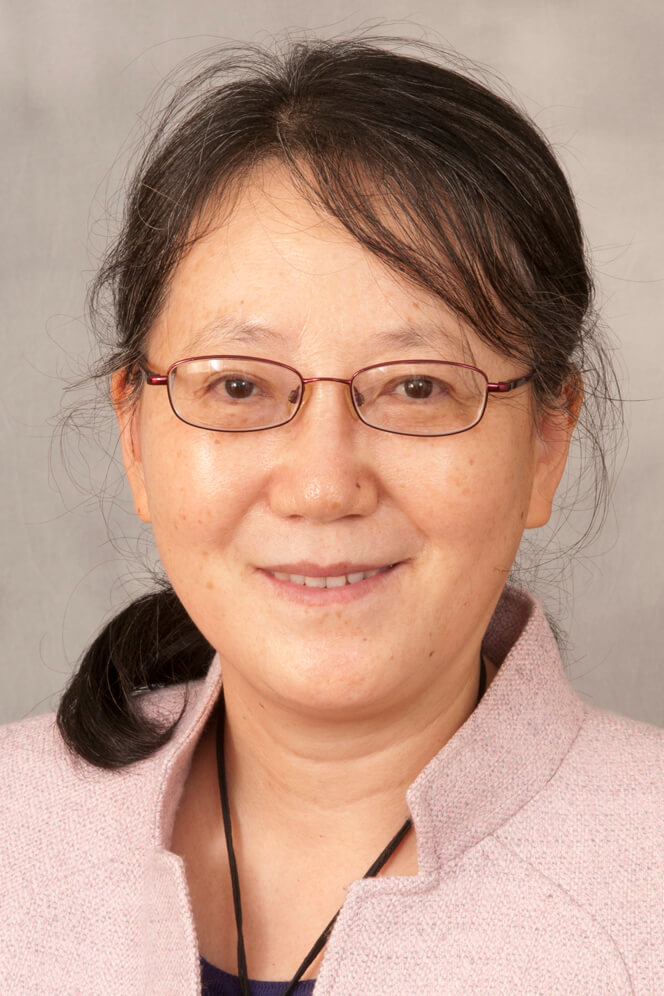# Betty Liu, Ph.D.

Professor

Department
Mathematics
Office
Howard Hall 239
Phone
732-571-4455
Email
bliu@monmouth.edu
Office Hours
On sabbatical Spring 2021

# Betty Liu, Ph.D.

## Education

Ph.D. in Applied Mathematics, University of Maryland, College Park

M.S. in Applied Mathematics, Nanging University, China

B.S. in Applied Mathematics, Nanging University, China

## Research Interests

My primary research area is numerical solutions of partial differential equations. My research interests are in the fields of numerical analysis, partial differential equations, scientific computation, mathematical modeling, and computational fluid dynamics. I have recently been concentrating on the following two fields of research: numerical analysis and computer simulation. For numerical analysis, I develop numerical methods to approximate the solutions of partial differential equations, prove the unique solvability of the numerical algorithms, and carry out the error analysis of the numerical solutions. For computer simulation, I develop 3D mathematical models to simulate the blood flows in human atherosclerotic arteries, to study the blood flow pattern in curved arteries with or without stenosis, and to investigate the effect of the stenosis on the wall shear stress, the pressure drop, and the flow disturbance. Numerical computations are carried out to allow for simulations of different geometries and flow parameters under the physiological conditions, and the numerical results are analyzed.

## Publications

### Scholarly Articles

A penalized finite element method for a compressible Stokes system, SIAM Journal of Numerical Analysis, Vol. 34, No. 3, pp. 1093-1105, 1997 (with B. Kellogg).

The analysis of a finite element method for the Navier-Stokes equations with compressibility, Numerische Mathematik, Vol. 87, pp. 153-170, 2000 (with B. Kellogg).

The analysis of a finite element method with streamline diffusion for the compressible Navier-Stokes equations, SIAM Journal of Numerical Analysis, Vol. 38, No. 1, pp.1-16, 2000.

On a finite element method for unsteady compressible viscous flows, Numerical Methods for Partial Differential Equations, Vol. 19, No. 2, pp. 152-166, 2003.

The influences of stenosis on the downstream flow pattern in curved arteries, Medical Engineering & Physics, Vol. 29, No., pp 868-876, 2007.

An error analysis of a finite element method for a system of nonlinear advection-diffusion-reaction equations, Applied Numerical Mathematics, Vol. 59, No. 8, pp. 1947-1959, 2009.

Influence of non-Newtonian properties of blood on the wall shear stress in human atherosclerotic right coronary arteries, Molecular & Cellular Biomechanics, Vol. 8, No. 1, pp.73-90, 2011 (with D. Tang).

Influence of model boundary conditions on blood flow patterns in a patient specific stenotic right coronary artery, BioMedical Engineering Online, Vol. 14 (Suppl 1):S6, pp.1-17, 2015 (with J. Zheng, R. Bach and D. Tang)

Influences of Flow Parameters on Pressure Drop in a Patient Specific Right Coronary Artery with Two Stenoses, O. Gervasi et al. (Eds.): ICCSA 2017, Part I, LNCS 10404, Springer. pp. 56–70, 2017 (with J., Zheng, R. Bach, and D. Tang)

Influence of the distal stenosis on the blood flow through coronary serial stenoses: A numerical study, International Journal of Computational Methods, Vol. 16, No. 3 (2019), 1842003, DOI: 10.1142/S0219876218420033 (with D. Tang).

## Courses

### Frequently Taught Classes

• Calculus for the Biological Sciences (MA 116)
• Calculus With Analytic Geometry I (MA 125)
• Calculus With Analytic Geometry II (MA 126)
• Calculus With Analytic Geometry III (MA 225)
• Differential Equations (MA 311)
• Introduction to Mathematical Modeling (MA 419)
• Introduction to Mathematics Modeling in the Biological Sciences (MA 115)
• Mathematical Modeling in the Social Sciences (MA 105)
• Pre-Calculus Mathematics (MA 109)